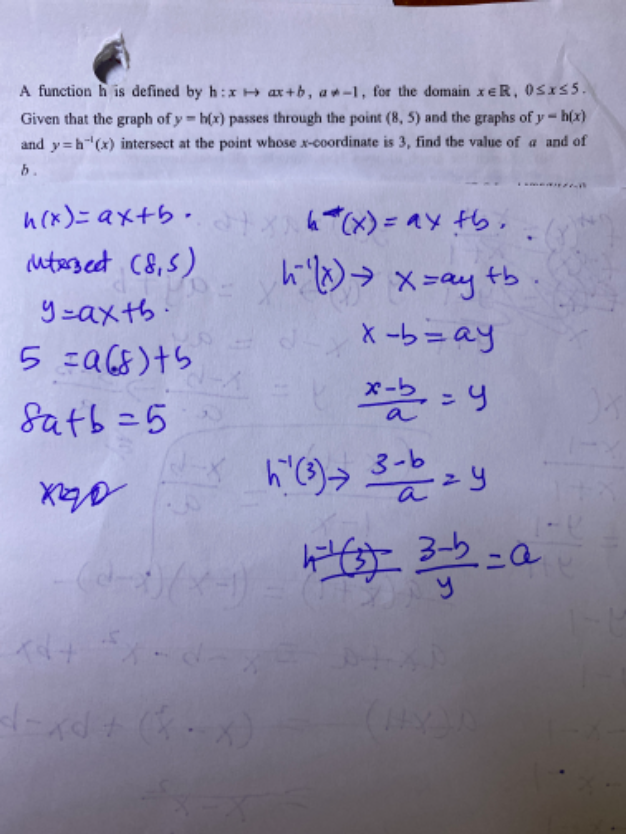# Questionb = 5-8a   ——– (1)

h(3) = 3a+b

h-1 (3) = (3-b)/a

Given h(3) = h-1 (3)

3a+b = (3-b)/a    => 3a2 +ab +b-3 = 0     ——-(2)

Substitute (1) into (2),

3a2  + a(5-8a) + (5-8a) -3 = 0

3a2  + 5a – 8a2 + 5 – 8a – 3 = 0

-5a2 -3a + 2= 0

5a2 + 3a – 2 = 0

(5a – 2) (a + 1) = 0

=> a = 2/5  or  a = -1  but it is given that a <> -1

Hence a = 2/5 =0.4 ,   b = 5-8(2/5) =  (9/5) or write it in mixed number 1  4/5  or  1.8